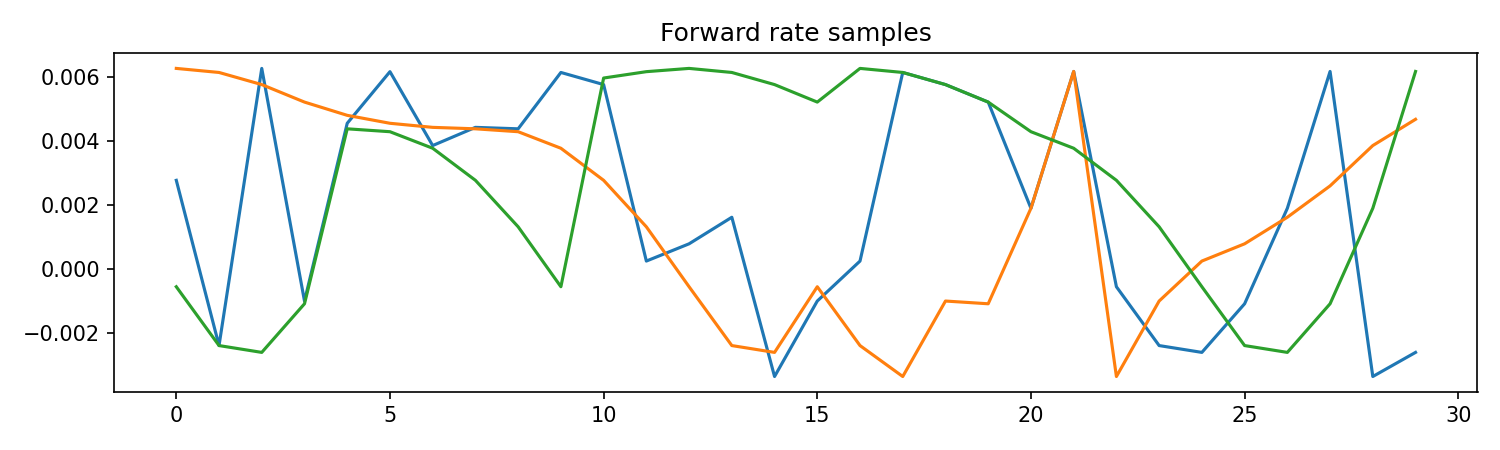# Example of the stationary bootstrap algorithm in practice#

:

import numpy as np
import pandas as pd
import matplotlib.pyplot as plt
%matplotlib notebook


## Goal#

The goal of this workbook is to demonstrate the use of the stationary bootstrap algorithm. In this example, 3 new samples are generated.

:

nSample = 3 # Number of generated samples


EURO denominated 6M interest-rate-swap rate obtained from https://www.teleborsa.it/Quotazioni/Tassi/Eurirs for date 12/11/2021 and interpolated using the Smith & Wilson algorithm for missing durations.

:

swap = pd.DataFrame({'Swap rate': [-0.00497999999999998,
-0.00336999999999998,
-0.00219000000000003,
-0.00137999999999994,
-0.000839999999999952,
-0.000349999999999961,
0.000140000000000029,
0.000669999999999948,
0.00116999999999989,
0.00165999999999999,
0.00208999999999993,
0.00245999999999991,
0.00277690952371801,
0.00303541131903184,
0.00323000000000007,
0.00336225372994803,
0.00345212702349018,
0.00351682745133952,
0.00356726077755898,
0.00360999999999989,
0.00364393467821089,
0.00365001038370538,
0.00360991273768496,
0.00350996855727703,
0.00333999999999990,
0.00310968998405592,
0.00288888447388769,
0.00274107227669318,
0.00271077735186531,
0.00283000000000011]})

:

swap.head()

:

Swap rate
0 -0.00498
1 -0.00337
2 -0.00219
3 -0.00138
4 -0.00084
:

sampleLen = swap.size; # Length of the sample will be the same as the input.


The swap rates cann ot be stacked in a sampling algorithm therefore, they are used to Vector with the 1-year forward curve. Convert rates into 1-year forward curve

Note that you loose 1 degree of freedom when converting from rates to 1-year-forward rates using the formula

$fwd(t) = \frac{(1 + r_{SWAP}(0, t+1))^{t+1}}{(1 + r_{SWAP}(0, t))^{t}}$
:

forwards = np.zeros((sampleLen-1,1))

:

for iTime in range(0,sampleLen-1):
forwards[iTime] = np.power(1 + swap["Swap rate"][iTime+1],iTime+1) / np.power(1+swap["Swap rate"][iTime], iTime) - 1

:

forwards = pd.DataFrame(forwards, columns={'Forward rate'})

:

forwards.head()

:

Forward rate
0 -0.003370
1 -0.001009
2 0.000242
3 0.000782
4 0.001612

Calculated using the method presented in 2004 paper by Politis & White.

:

m = 3.8299


Generate Stationary bootstraped samples

In this step, the samples are generated by repeatedly calling th StationaryBootstrap function. These samples are then saved into the Samples array

:

from StationaryBootstrap import StationaryBootstrap
for iSample in range(0, nSample):
if iSample == 0:
Samples = np.array(StationaryBootstrap(forwards.values,m,sampleLen))
else:
Samples = np.append(Samples, np.array(StationaryBootstrap(forwards.values,m,sampleLen)),axis=1)

:

Samples = pd.DataFrame(Samples)

:

0 1 2
0 0.002768 0.006270 -0.000561
1 -0.002402 0.006143 -0.002402
2 0.006270 0.005763 -0.002615
3 -0.001009 0.005216 -0.001094
4 0.004553 0.004801 0.004380
:

plot_data = Samples.copy().to_numpy()
fig, ax = plt.subplots(figsize=(10, 3))
ax.plot(plot_data)
ax.set_title('Forward rate samples')
fig.tight_layout()